# 精准用户画像！商城用户分群2.0！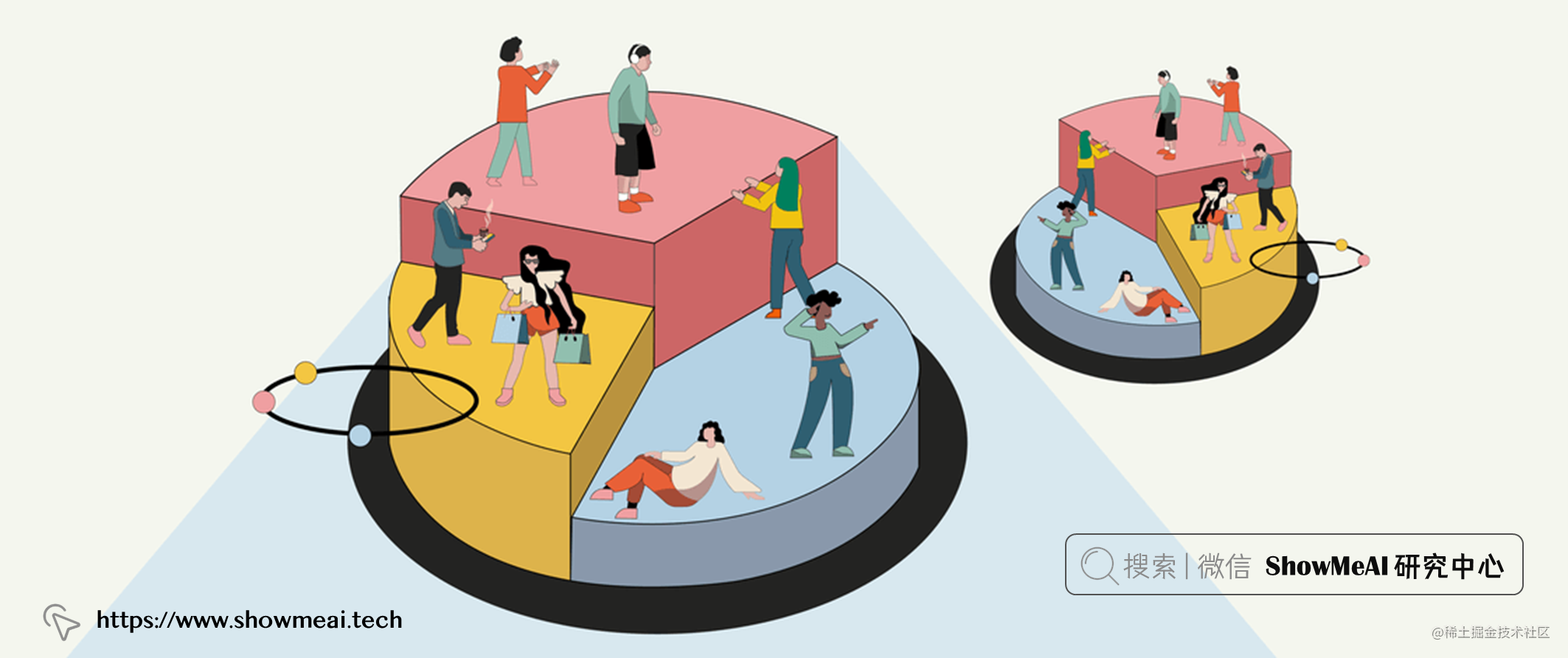# 💡 数据加载 & 基本处理

🏆 实战数据集下载（百度网盘）：公众号『ShowMeAI研究中心』回复『实战』，或者点击 这里 获取本文 基于多种聚类算法的商城用户分群！绘制精准用户画像Mall_Customers数据集

ShowMeAI官方GitHubgithub.com/ShowMeAI-Hu…

``````df= pd.read csv( "Mall Customers.csv")
df.rename (columns={"CustomerID": "id", "Age": "age", "Annual Income (k\$)": "annual_income", "Spending Score (1-100)": "spending_score"}, inplace=True)
df.drop(columns=["id"], inplace=True)

# 💡 探索性数据分析``````numcol = ["age", "annual_income", "spending_score"]
objcol = ['Gender']

## 💦 单变量分析

### ① 类别型特征

``````sns.set_style("ticks")
my_pal = {"Male": "slateblue", "Female": "lightsalmon"}
ax = sns.countplot(data=df, x="Gender", palette=-my_pal)
ax.grid(True, axis='both' )
for p in ax.patches:
ax.annotate( '{:.Of}'. format(p.get _height()), (p.get _x()+0.25, p.get_height()+0.3))
percentage = "{:.If}%'. format(100 * p.get height )/lendf[ "Gender" ]))
ax.annotate(percentage, (p.get x()+0.25, p.get height ( )/2))
olt.title( "Gender Countolot")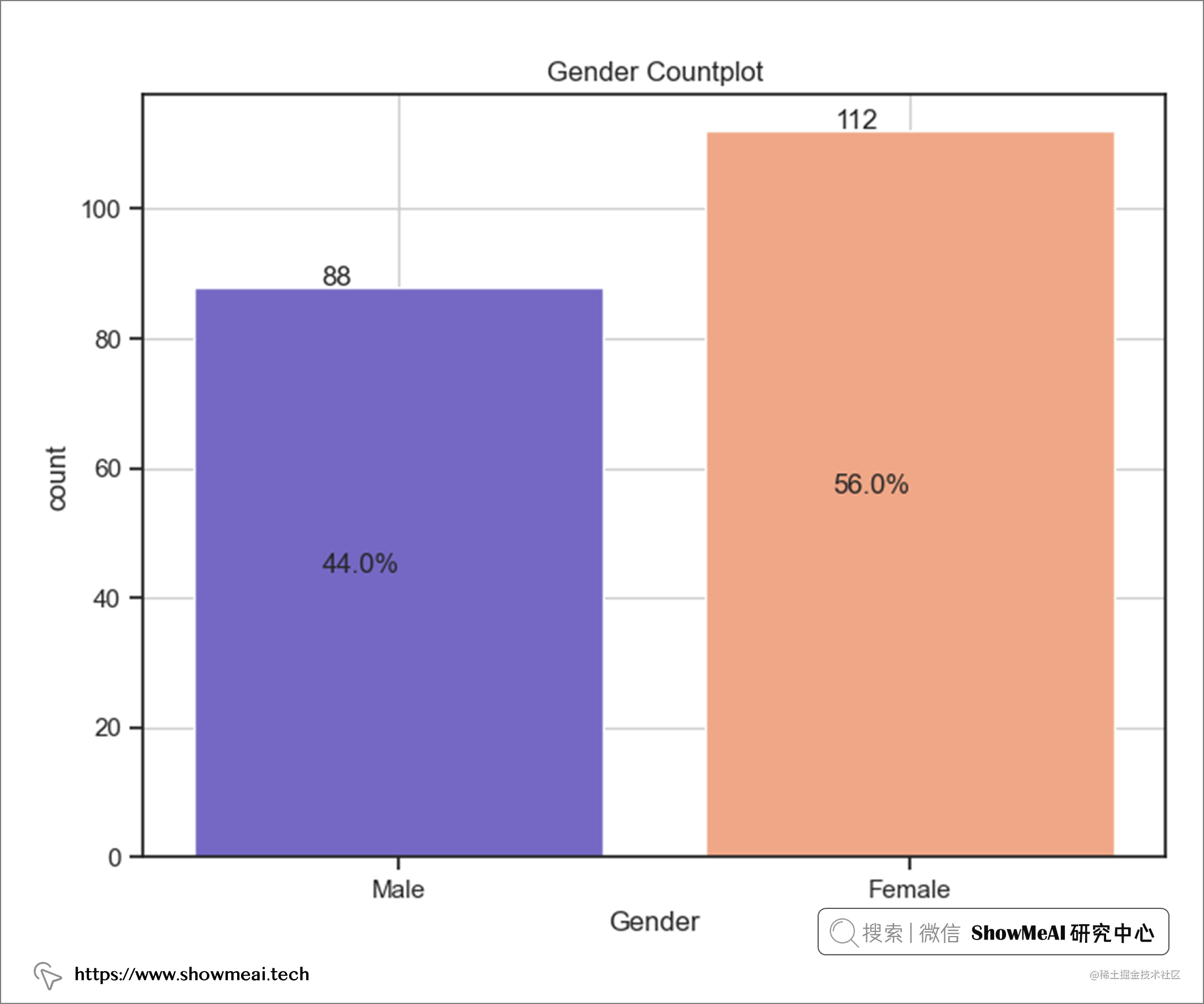### ② 数值特征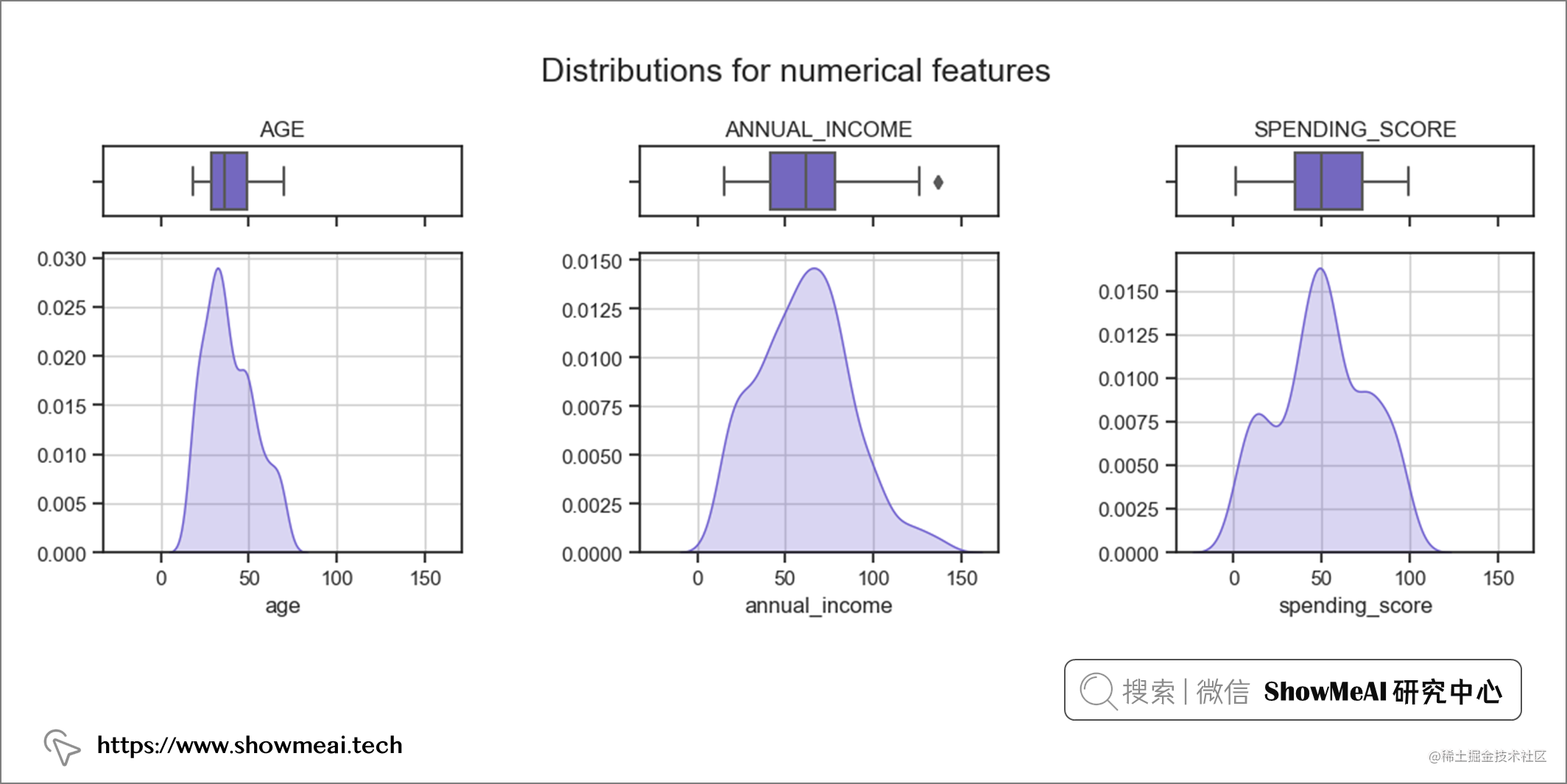``````sns.set_style("ticks", {'axes.grid' : False})
for idx, col in enumerate (numcol):
plt.figure()
f, ax = plt.subplots(nrows=2, sharex=True, gridspec_kw={"height_ratios": (0.2,0.85)}, figsize=(10,8));
plt.suptitle(f"{col.upper()}",y=0.93);
sns.boxplot(data=df,x=col,ax=ax,color="slateblue",boxprops=dict(alpha=.7),
linewidth=0.8, width=0.6, fliersize=10,
flierprops={ "marker" :"O", "markerfacecolor": "slateblue"},
medianprops={ "color": "black", "linewidth":2.5})

sns.histplot(data=df, ×=col, ax=ax,multiple="layer", fill=True, color= "slateblue", bins=40)
ax2 =ax.twinx()
sns.kdeplot(data=df, x=col, ax=ax2,
multiple="layer",
fill=True,
color="slateblue",
alpha=0.1,
linestyles="--")

ax.grid(False)
ax.set(xlabel="");

ax.set _xlabel(col, fontsize=14)
ax.grid(True)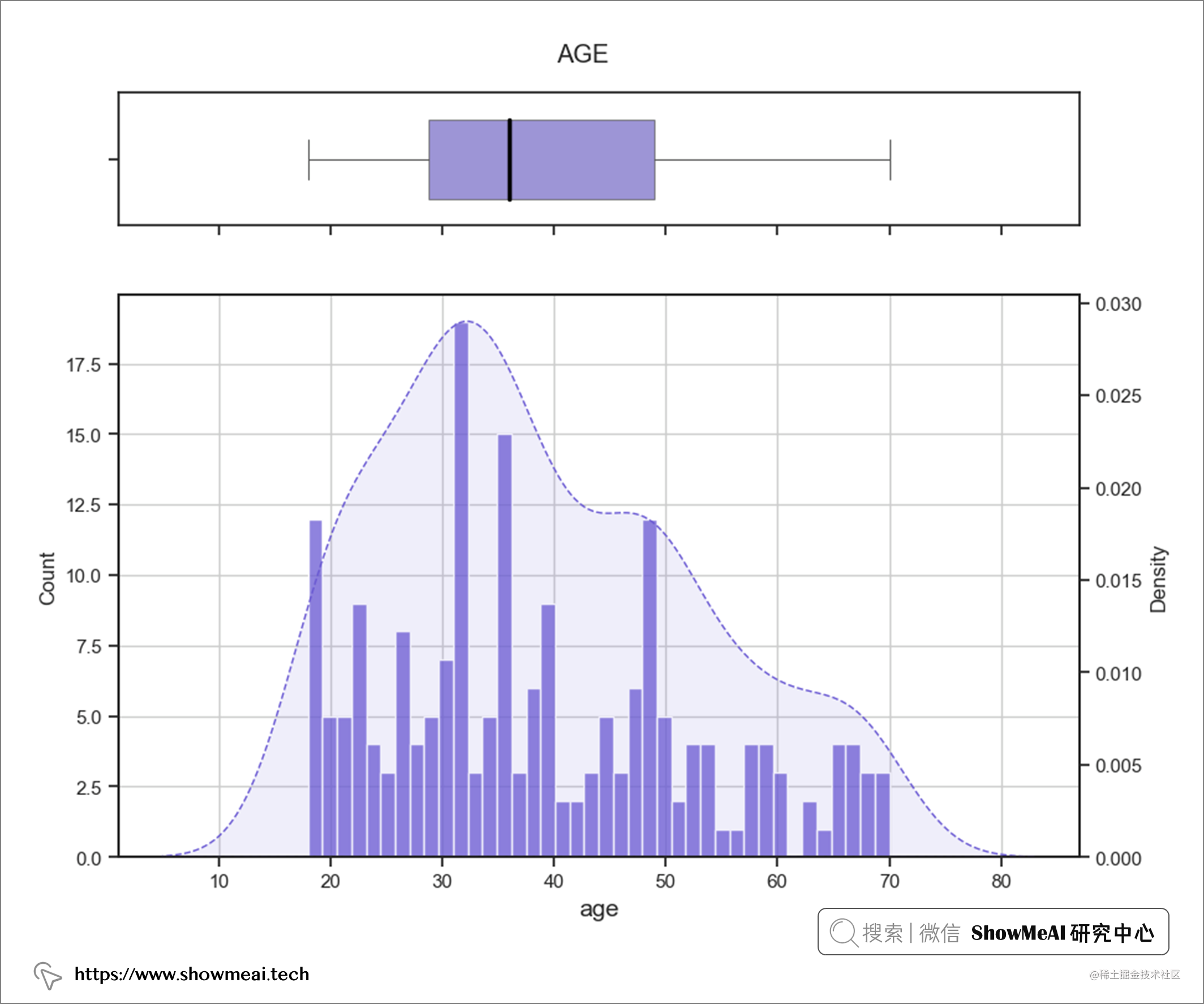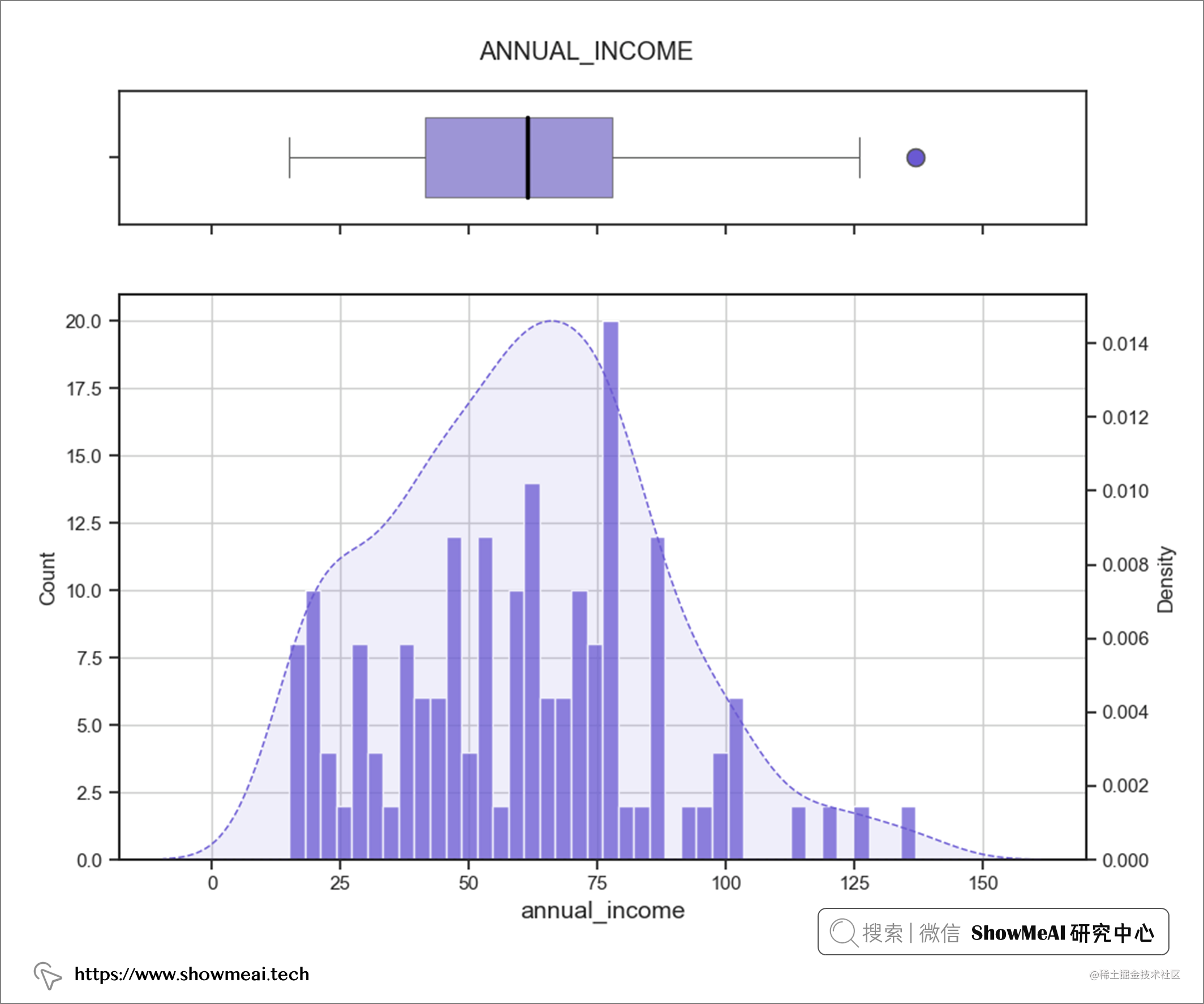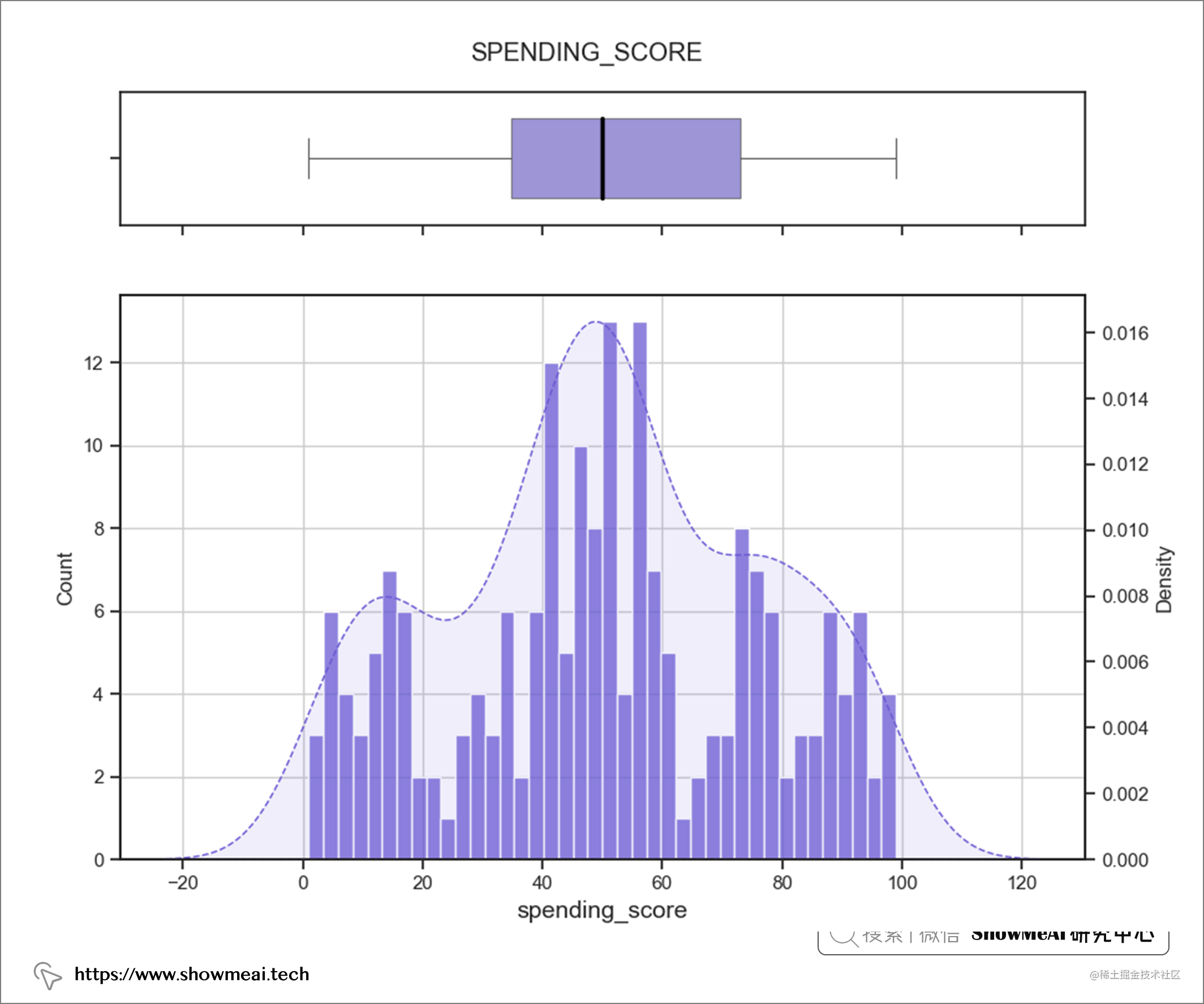## 💦 双变量分析

``````sns.set_style("ticks", {'axes.grid' : False})

def pairplot_hue(df, hue, **kwargs):
g = sns.pairplot(df, hue=hue, **kwargs)
g.fig.suptitle(hue)
return g

pairplot_hue(df[numcol+objcol], hue='Gender')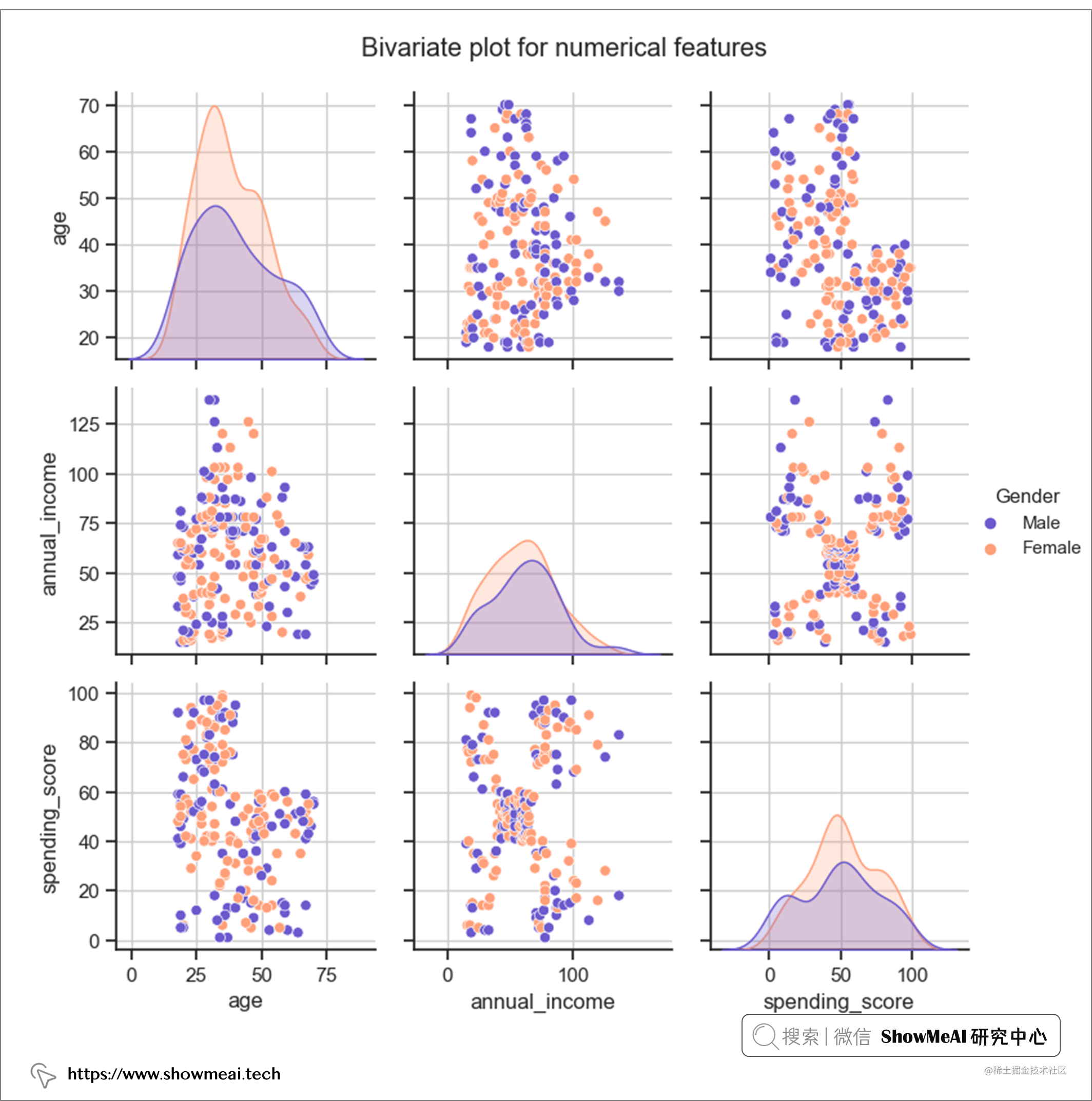# 💡 建模

## 💦 数据缩放

``````scaler = MinMaxScaler()
df_scaled = df.copy()
for col in numcol:
df scaled[col] = pd.DataFrame(scaler.fit_transform(df_scaled[col].values.reshape(-1,1) ))

## 💦 模型选择

### ① K-Means 聚类

K-Means 算法是一种无监督学习算法，它通过迭代和聚合来根据数据分布确定数据属于哪个簇。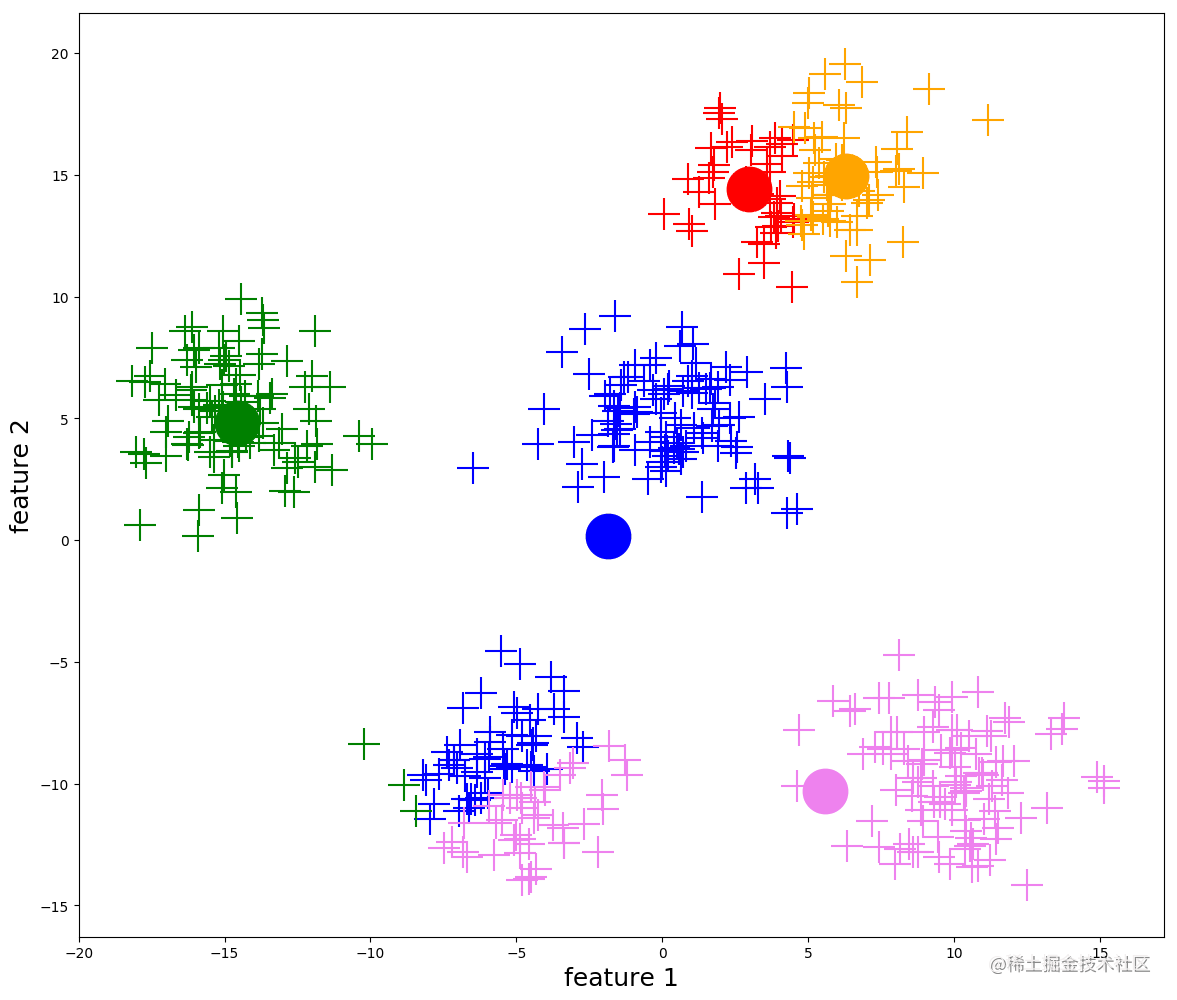### ② 层次聚类(BIRCH) 算法

BIRCH（Balanced Iterative Reducing and Clustering Using Hierarchies）翻译为中文就是『利用层次方法的平衡迭代规约和聚类』，全称非常复杂。简单来说，BIRCH 算法利用了一个树结构来帮助我们快速的聚类，这个特殊的树结构，就是我们后面要详细介绍的聚类特征树（CF-tree）。简单地说算法可以分为两步：

• 1）扫描数据库，建立一棵存放于内存的 CF-Tree，它可以被看作数据的多层压缩，试图保留数据的内在聚类结构；

• 2）采用某个选定的聚类算法，如 K-Means 或者凝聚算法，对 CF 树的叶节点进行聚类，把稀疏的簇当作离群点删除，而把更稠密的簇合并为更大的簇。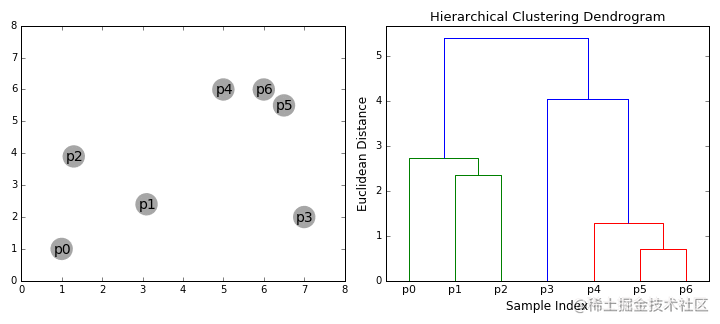## 💦 模型评估

### ① 聚类算法评估

◉ 轮廓分数（Silhouette score）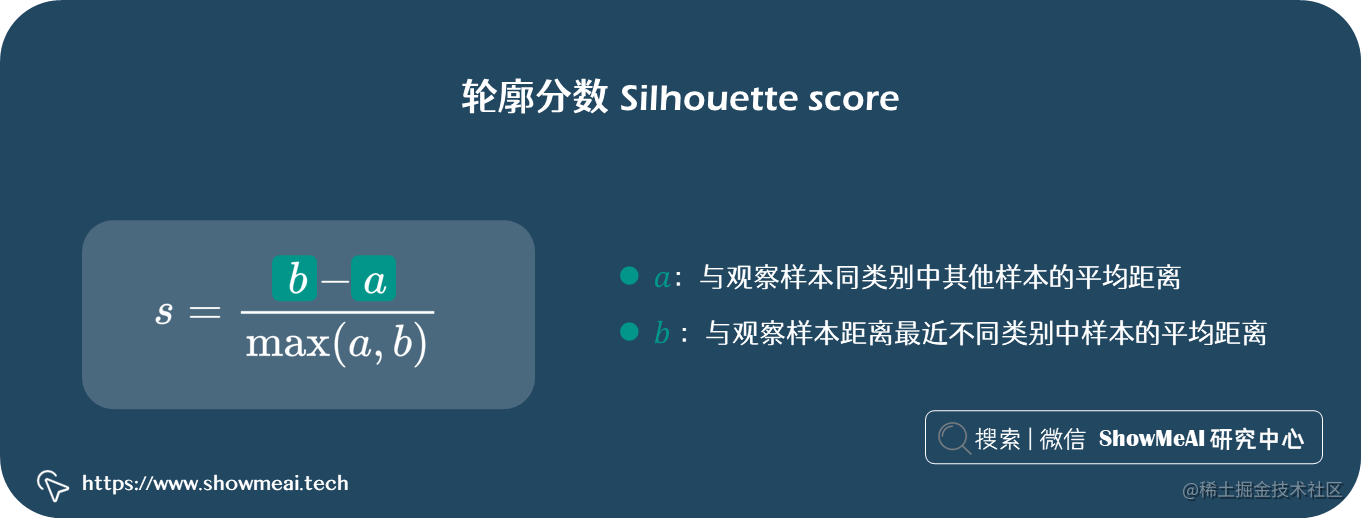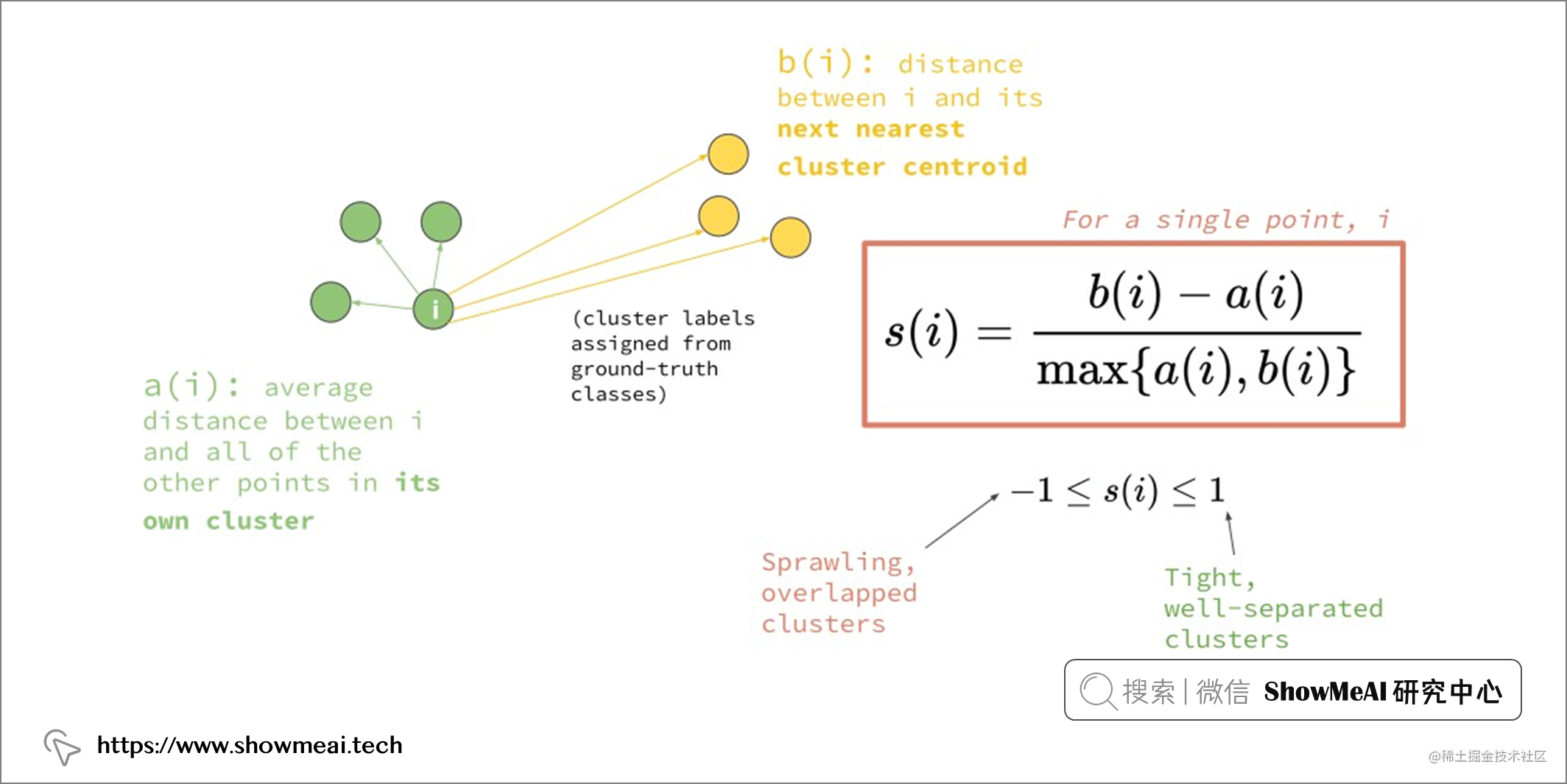◉ 卡林斯基哈拉巴斯得分（Calinski Harabasz score）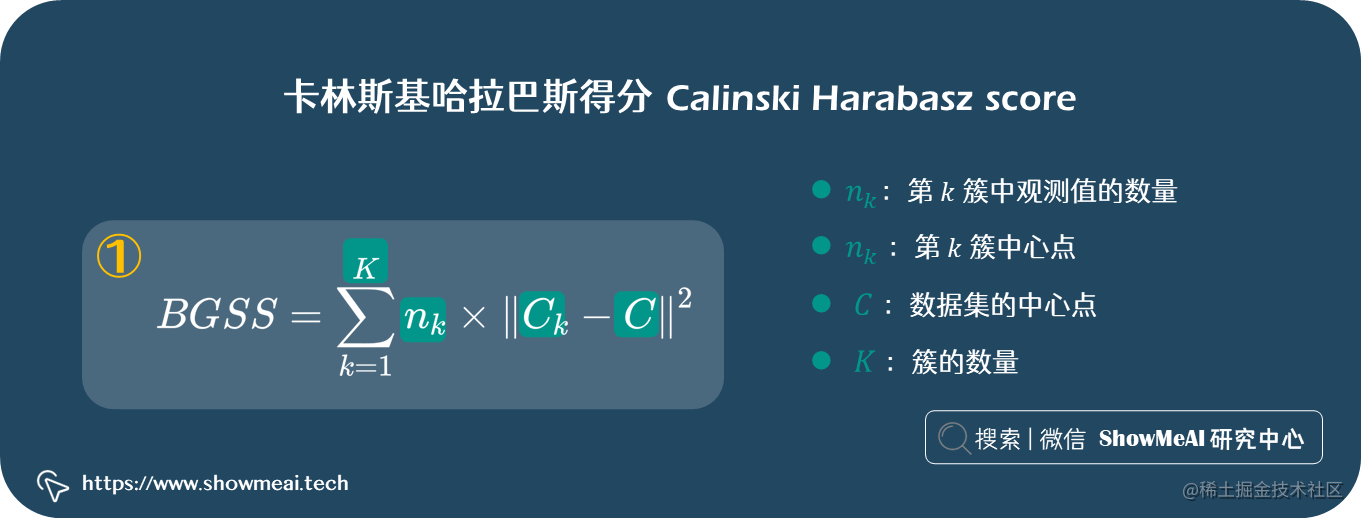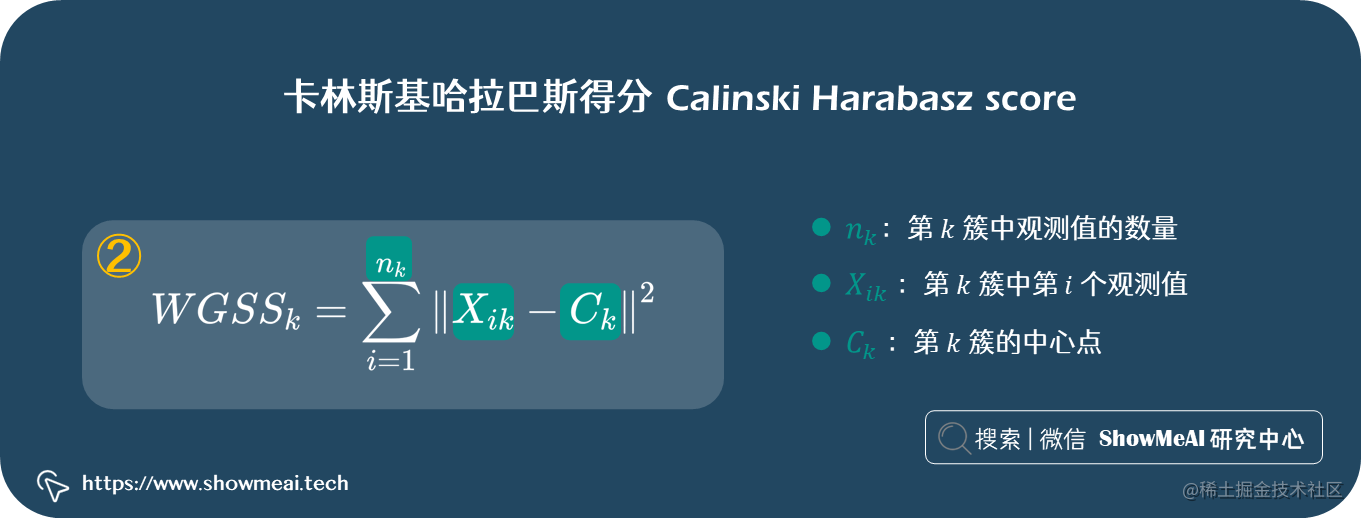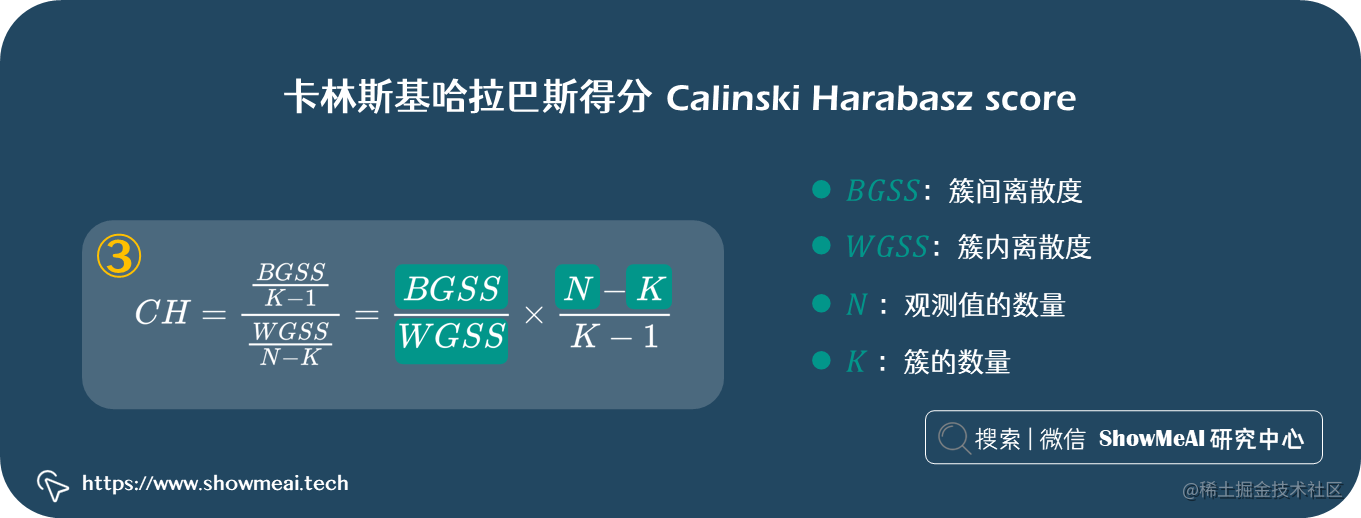◉ 戴维斯布尔丹得分（Davies Bouldin score）

### ② 应用 K-Means 聚类

``````k_range = range(2,10)
for x in k range:
model = KMeans(n_clusters=x, random_state=42)
X = df_scaled[[ "annual_ income", "spending_score"]]
model.fit(x)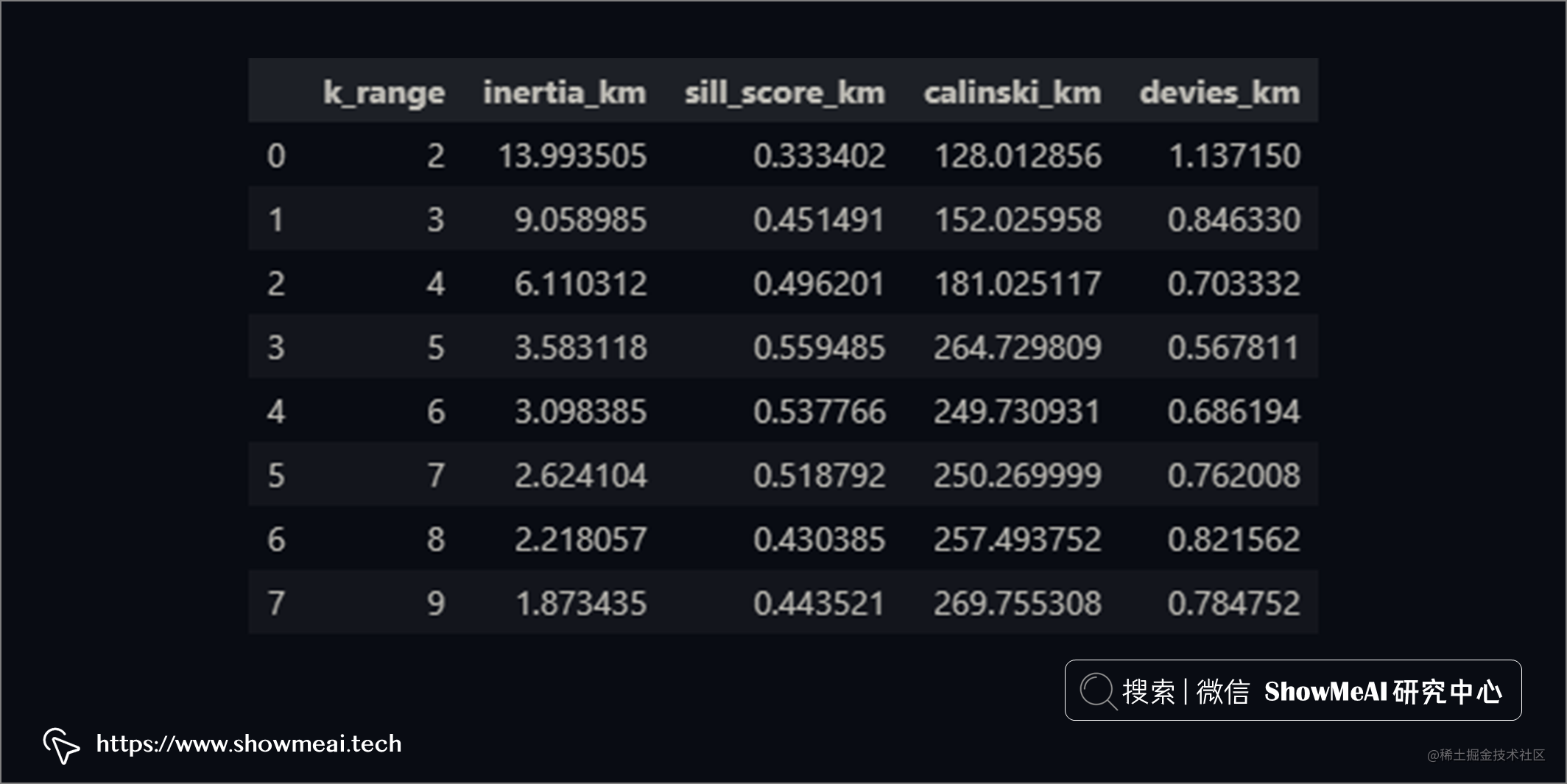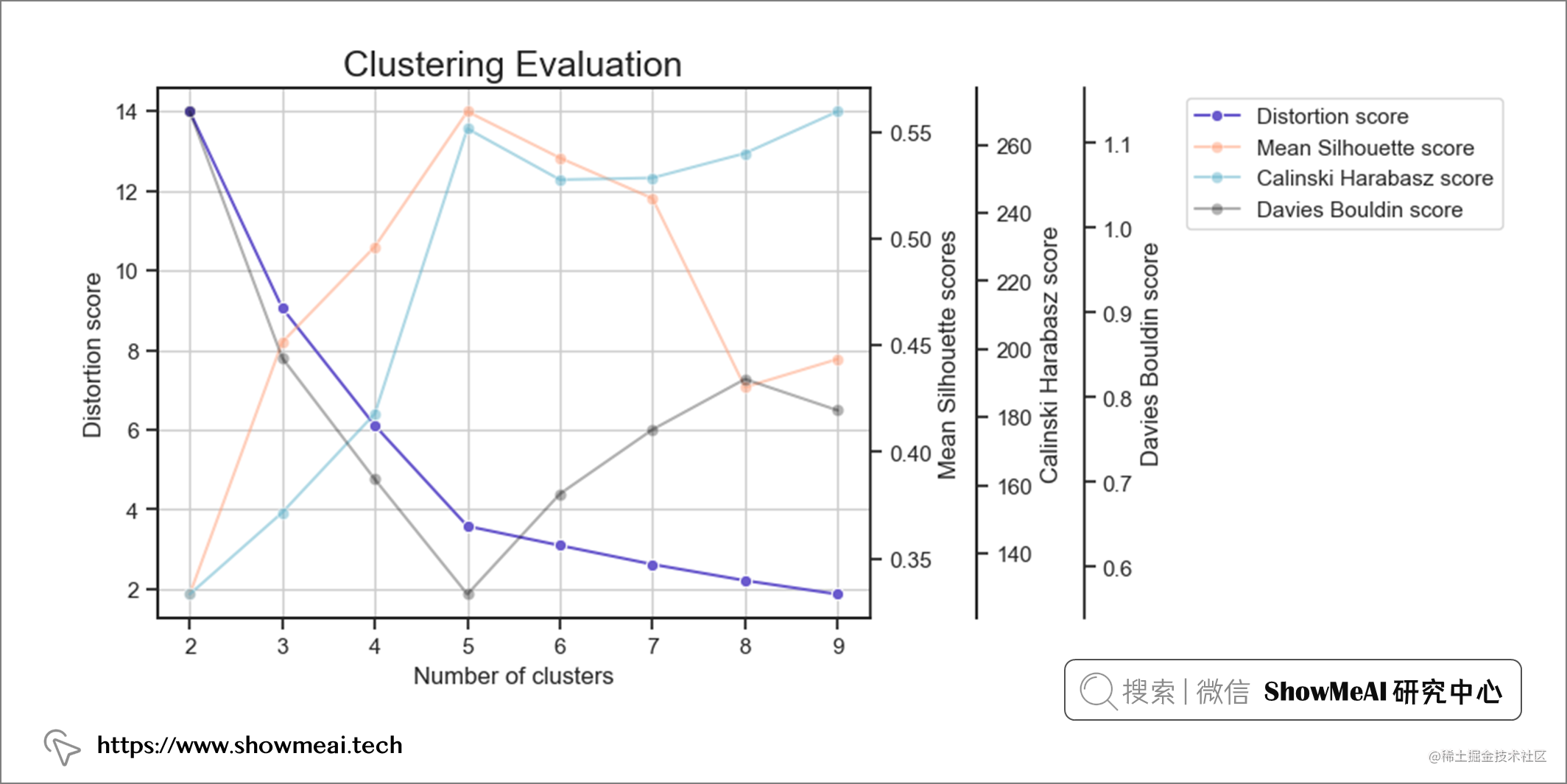• 开始递减收益（肘法）
• 最高平均轮廓分数
• 相对较高的 Calinski Harabarsz 评分（局部最大值）
• Davies Bouldin 最低分数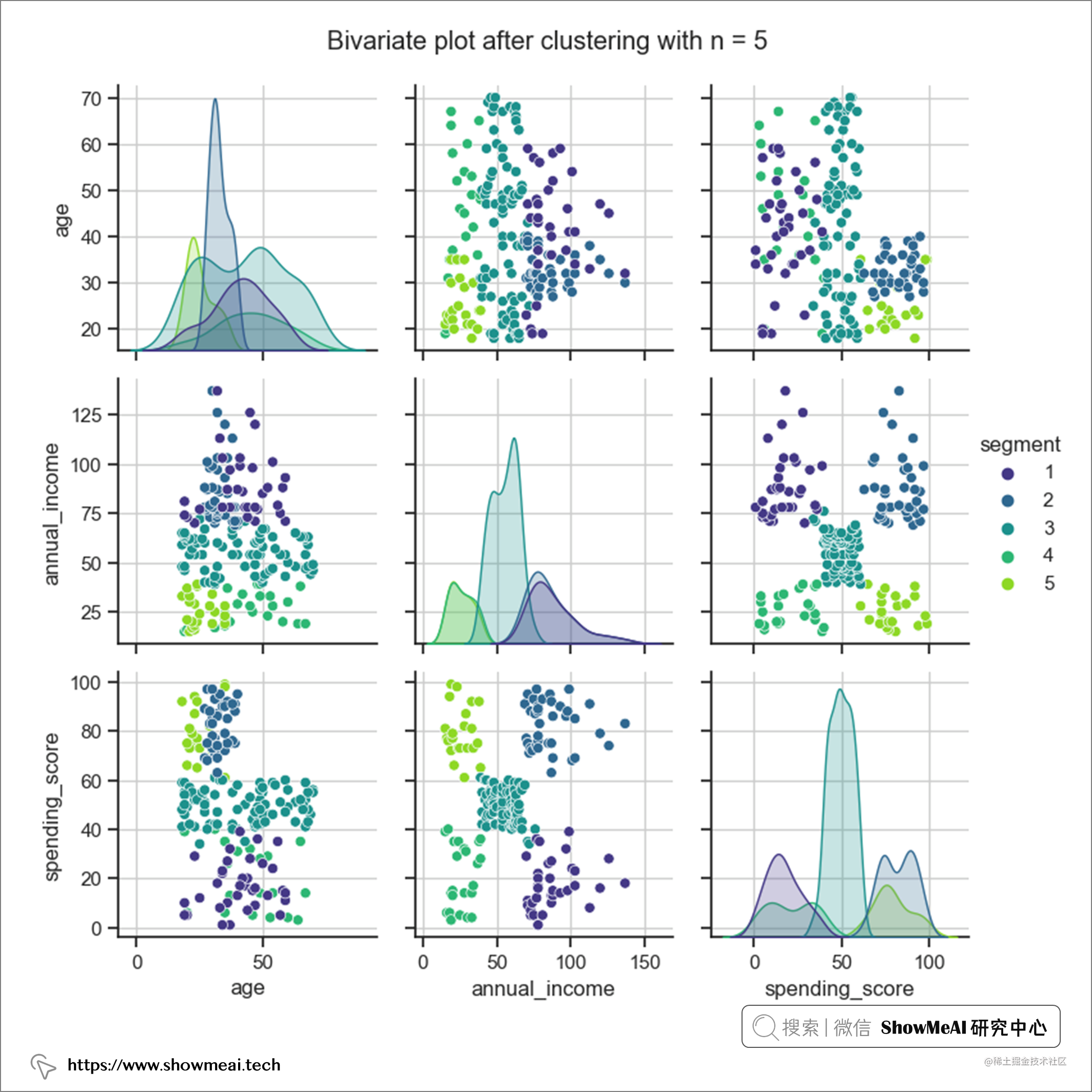### ③ 应用 BIRCH 聚类

``````n = range(2,10)
for x in n:
model = Birch(n_clusters=x, threshold=0.17)
X = df_scaledI[ "annual income", "spending_score"]]
model.fit(X)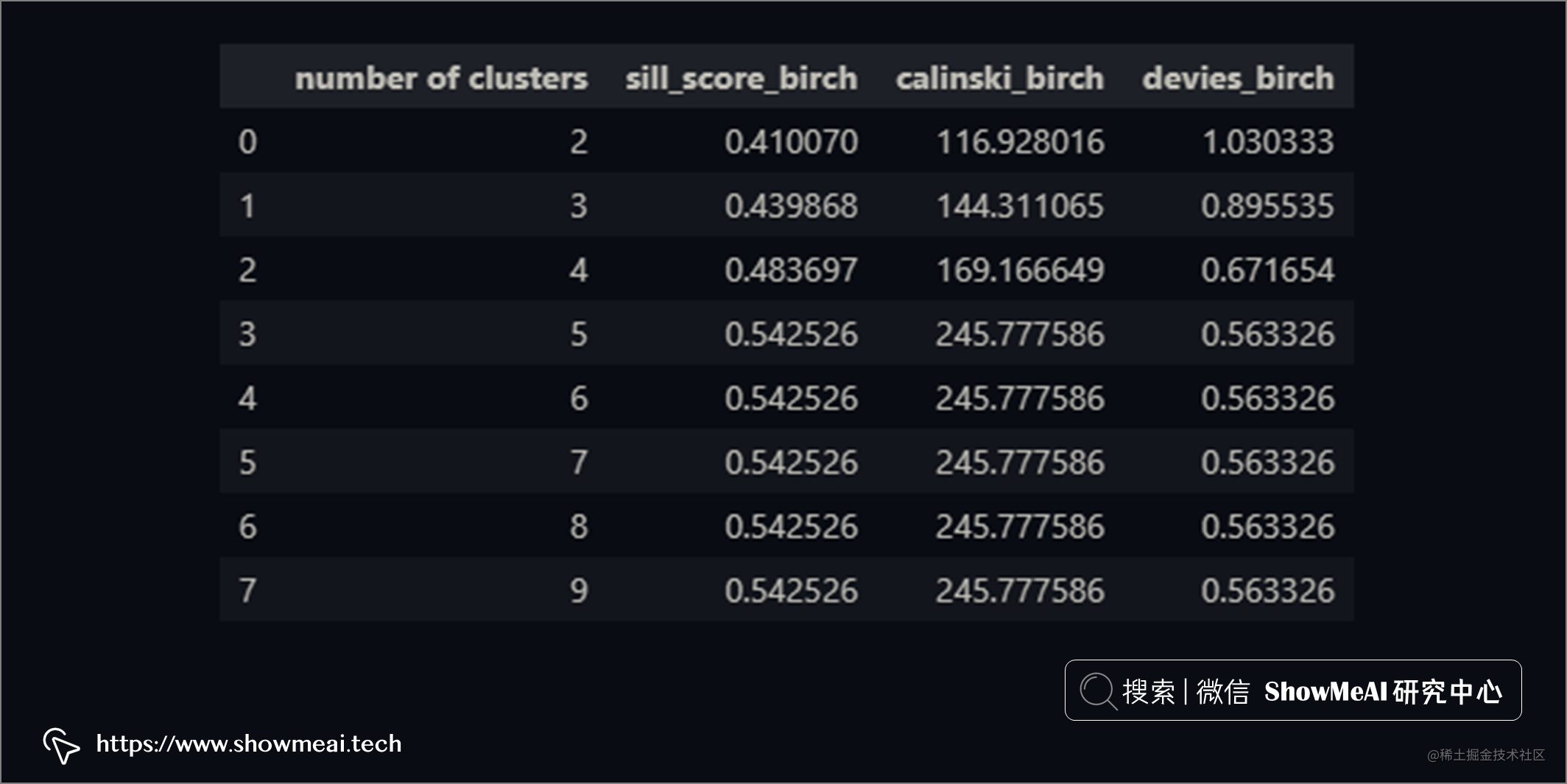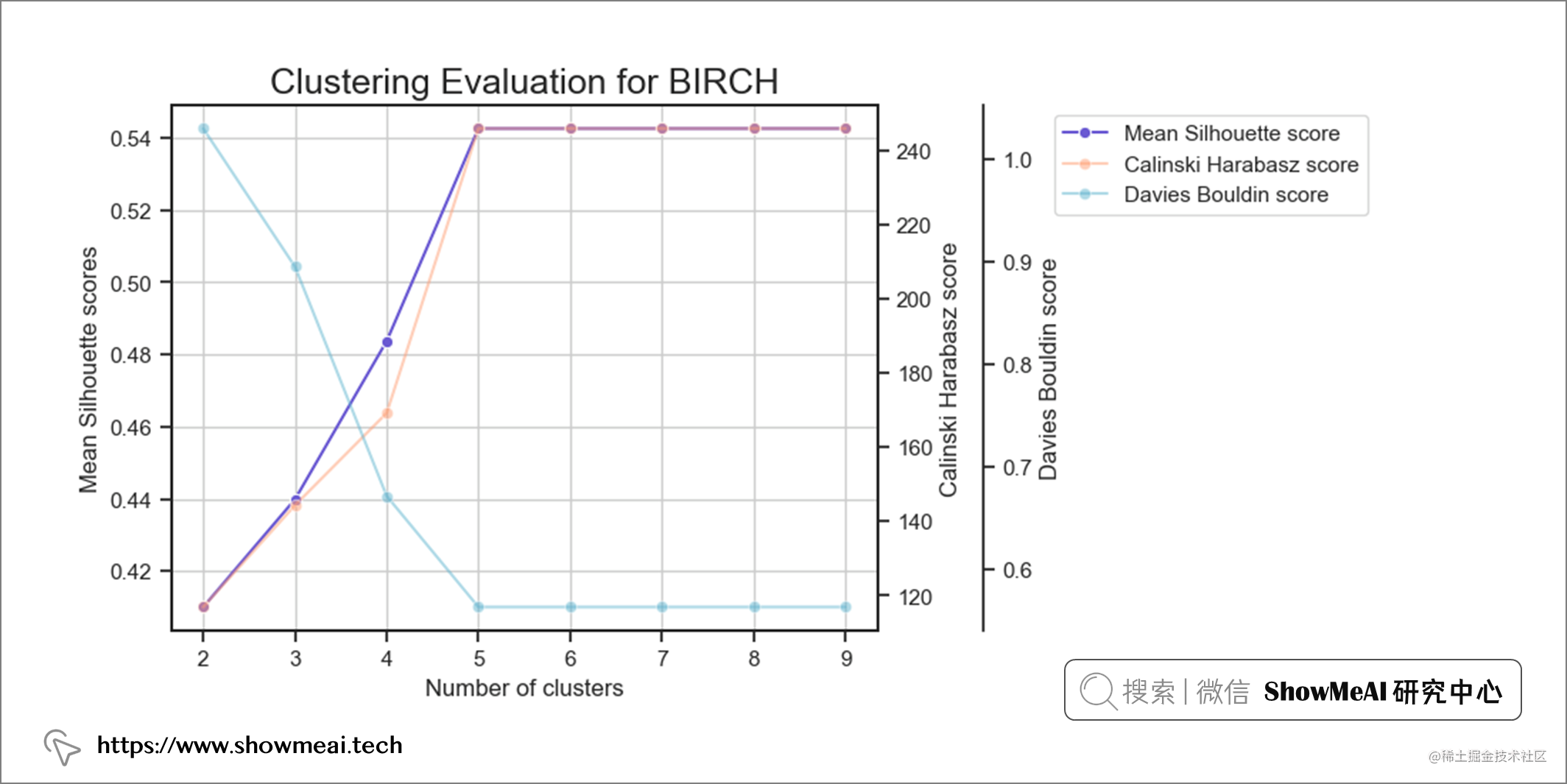BIRCH 的计算也给出了簇数等于5这样的一个结论。我们同样对数据进行分布分析绘图，不同的用户簇的数据分布如下（依旧可以比较清晰看到不同用户群的分布差异）。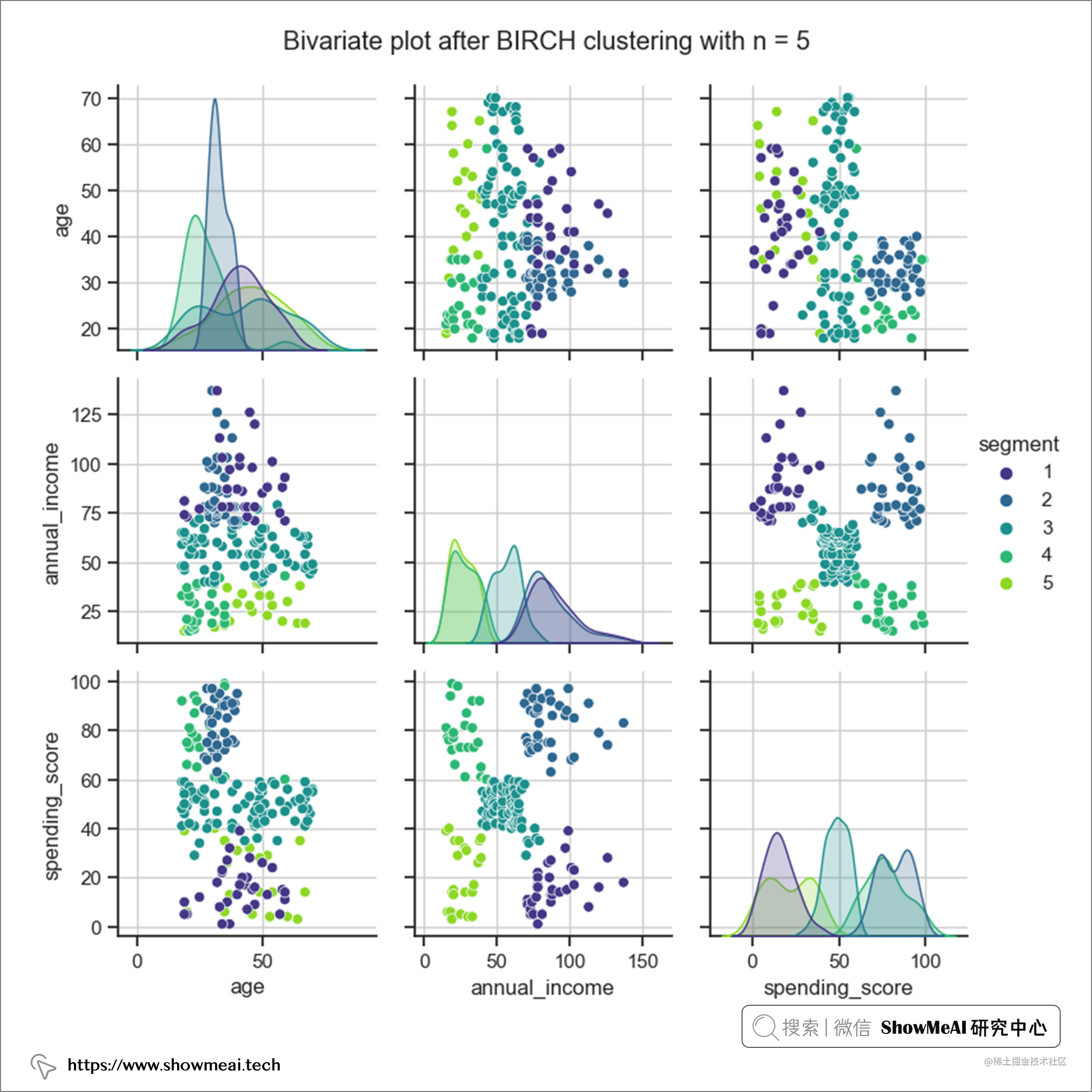### ④ 建模结果解释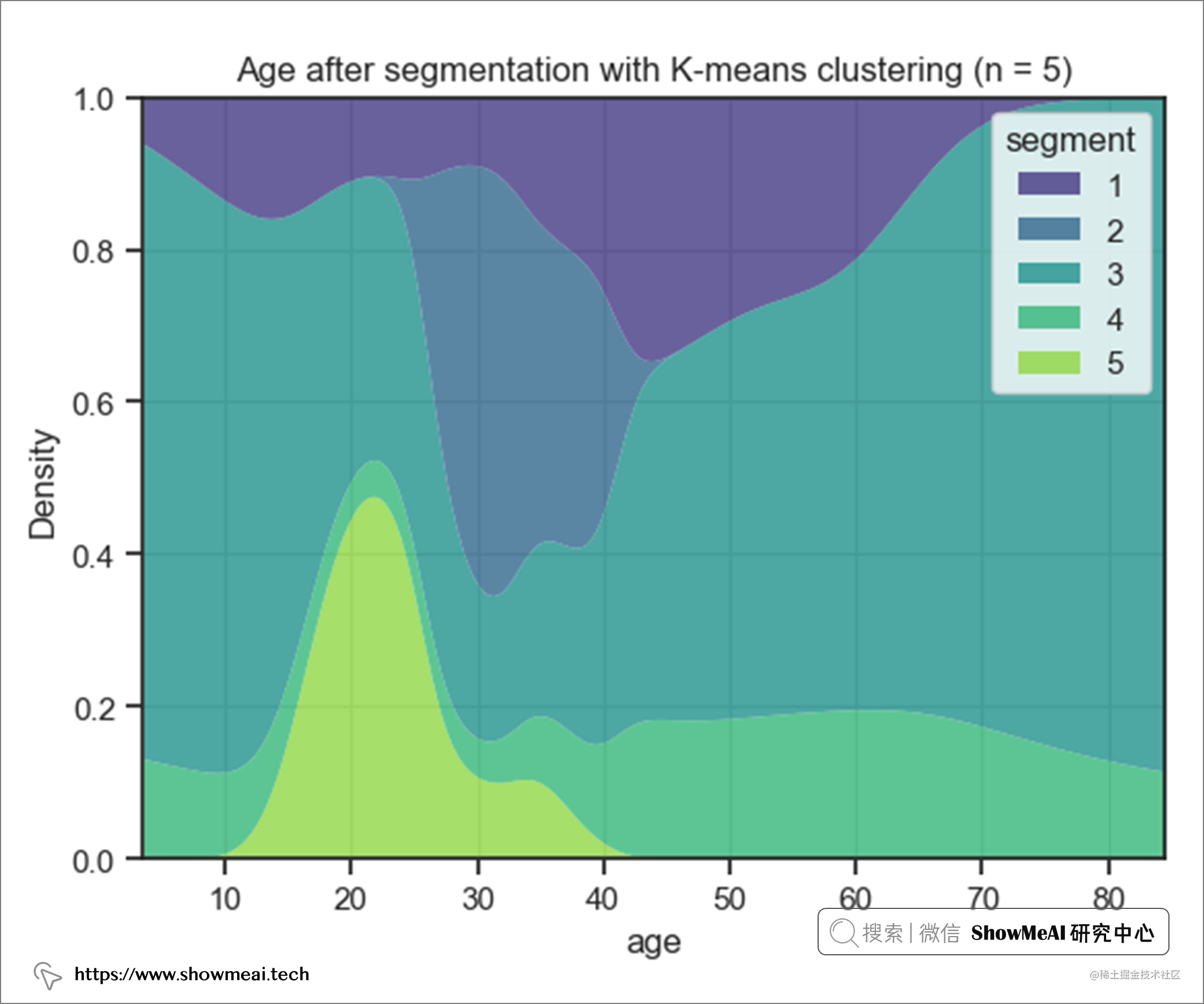• 第 2 个用户簇 => 年龄在 27 到 40 岁之间 ，平均值为 33 岁。
• 第 5 个用户簇 => 年龄在 18 到 35 岁之间 ，平均为 25 岁。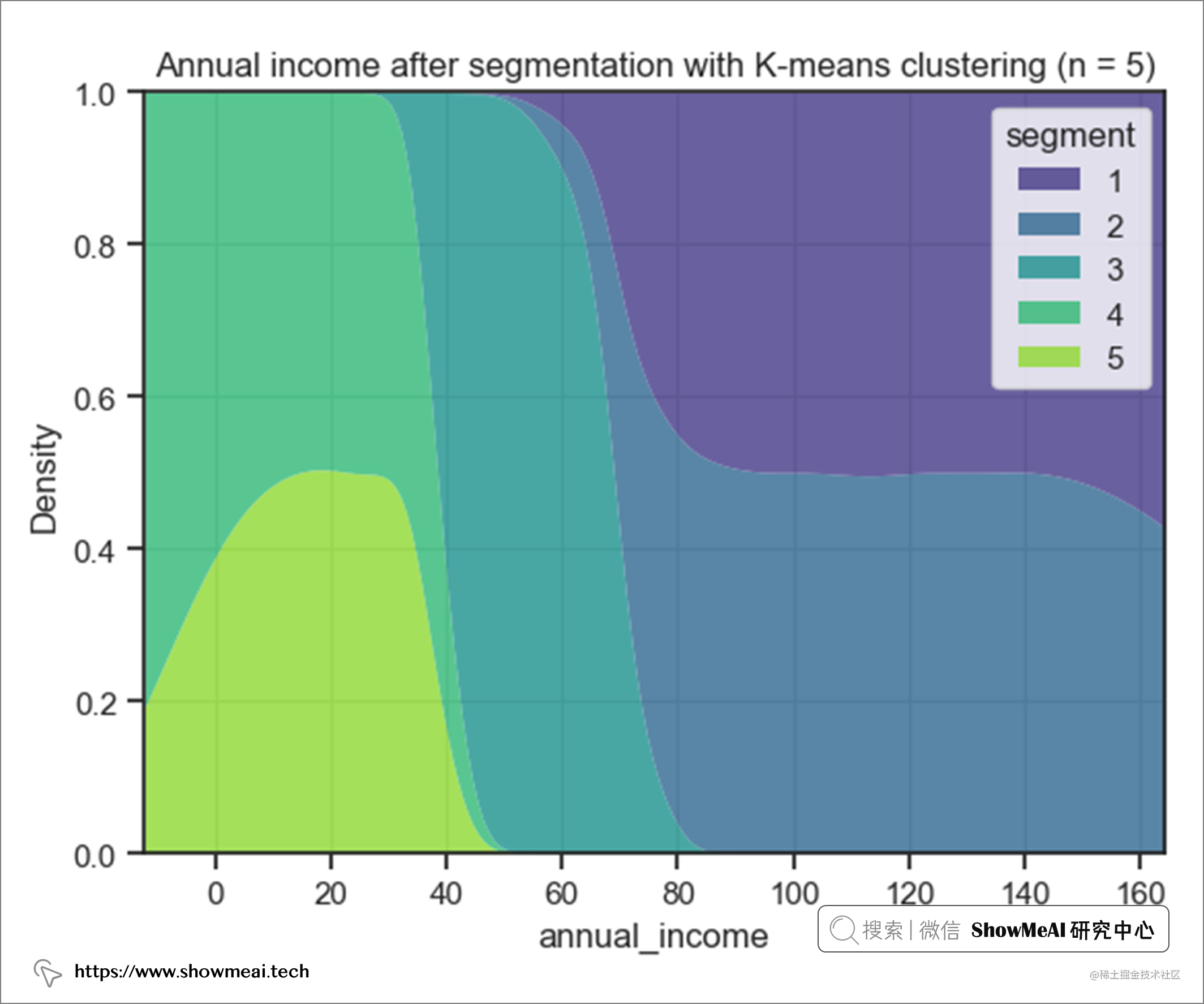• 用户群4和5的年收入大致相等，大约为 26,000 美元。 → 低收入群体
• 用户群1和2的年收入大致相等，这意味着大约 87,000 美元。 → 高收入群体
• 用户群3是独立组，平均年收入为 55,000 美元。 → 中等收入群体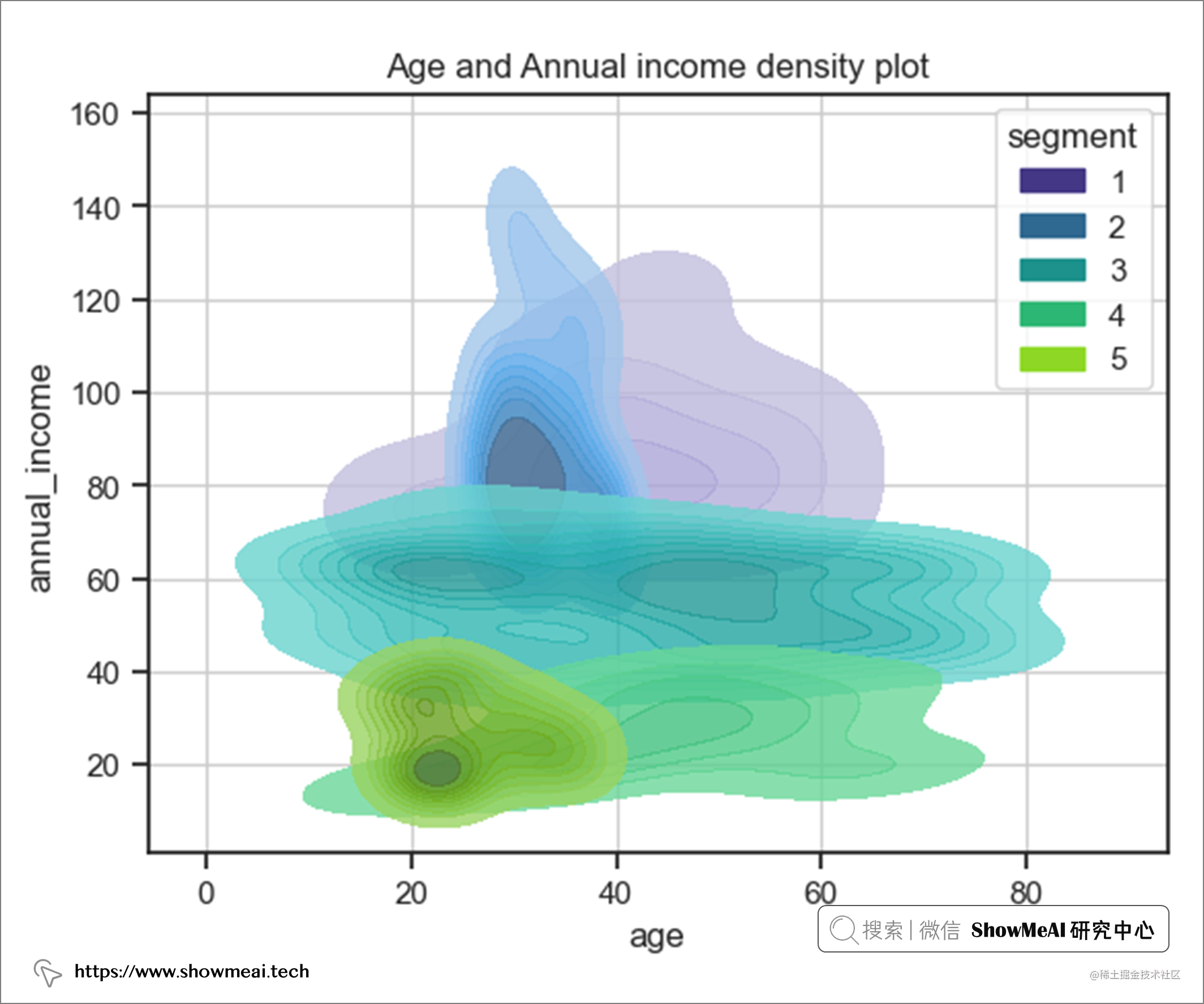• 用户群2和5的年龄范围相同，但年收入有显着差异
• 用户群4和5的年收入范围相同，但第 5 段属于青少年组（20-40 岁）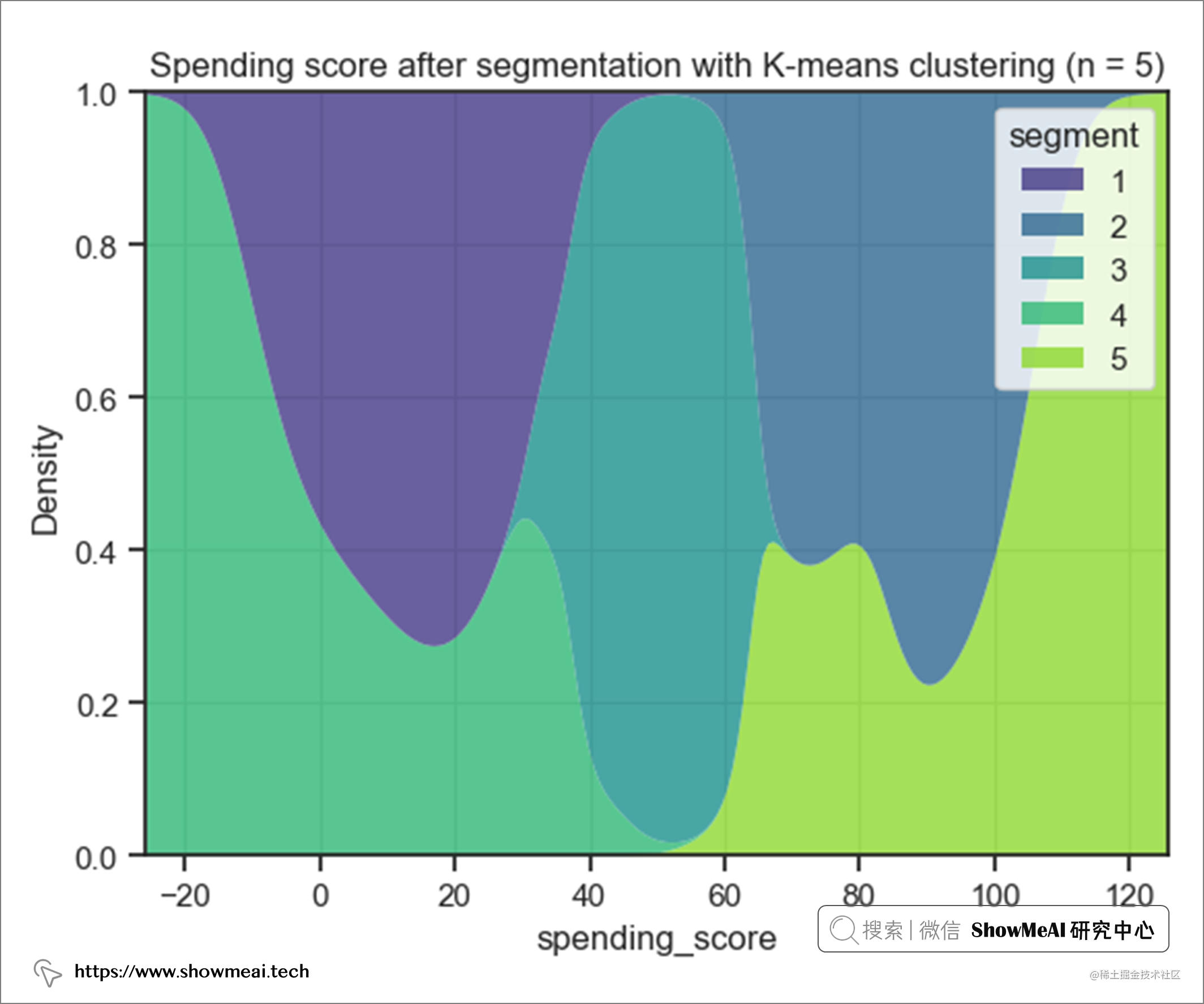• 用户群5的 支出得分最高
• 用户群4的 支出得分最低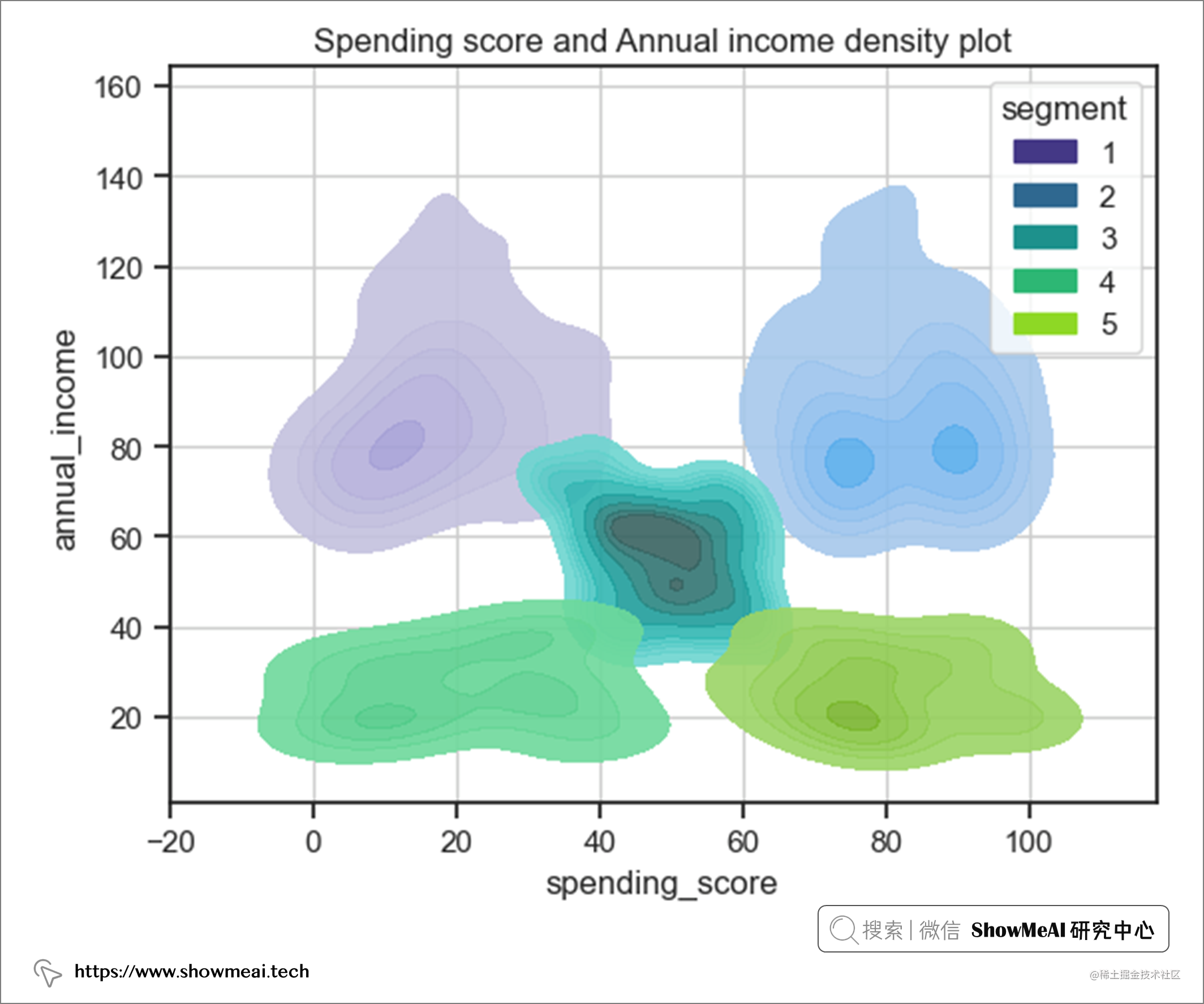• 用户群1和2的年收入范围相同，但支出分范围完全不同。
• 用户群4和5的年收入范围相同，但支出分范围完全不同。

# 💡 结论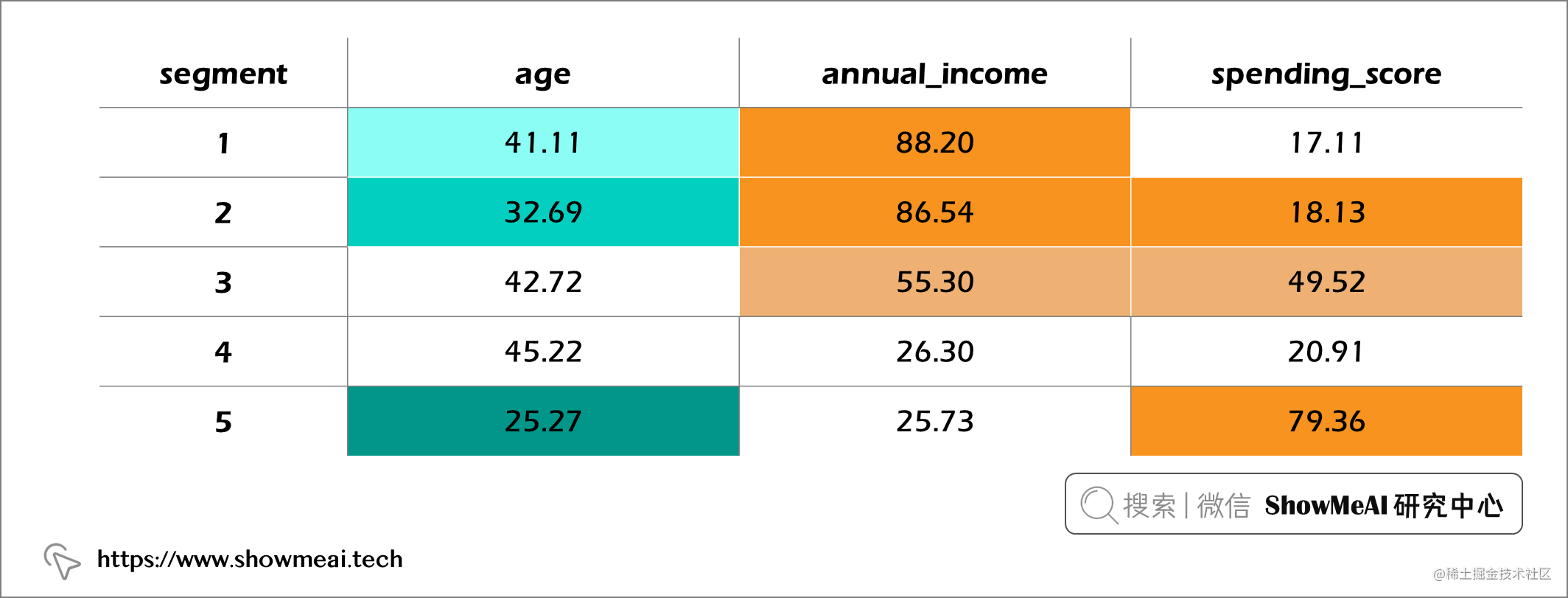• 用户群1是最高年收入组，但有最差的支出消费。 → 目前商城的产品并不是这部分客户的消费首选（非目标客户）。
• 用户群2的平均年龄比第 1 段低 10 倍，但在相同年收入范围内的平均支出分数是 4 倍。
• 用户群5是最高支出分数但是最低年收入组。 → 客户购买欲望强，但消费能力有限。

# 参考资料